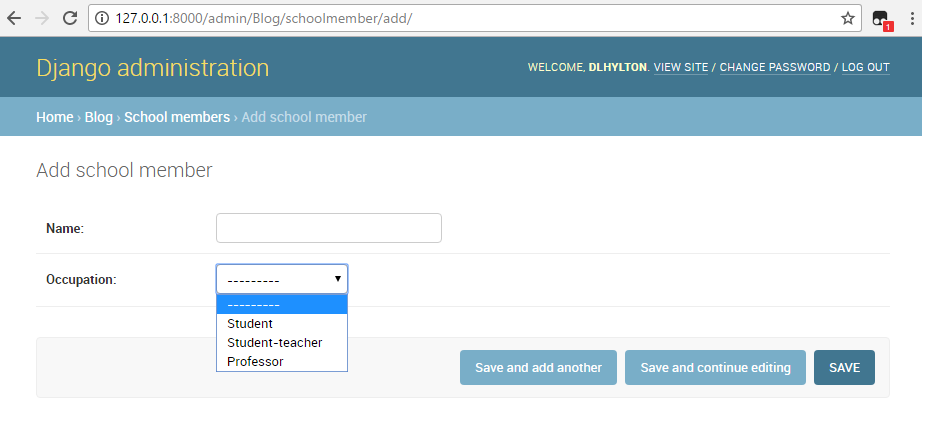﻿ How to Create a Database Table Field that Contains Choices in Django ﻿# How to Create a Database Table Field that Contains Choices in DjangoIn this article, we show how to create a database table field that contains choices in Django.

What is meant by this is we limit the response that can go inside of an actual database to certain choices. For example, if we have a database field that asks what occupation are you, student, student-teacher, or professor, we can limit these choices to these fields, so that only these 3 choices can be added to the database.

So, how can this be done?

So we can do this by adding a keyword called choices inside of the field, in which we can specifiy the choices usually through a list of tuples (or tuple of tuples, doesn't matter).

We show how to do this below.

Below we create a database table called SchoolMember.

We ask for 2 fields, the person's name and the person's occupation within the school. With the occupation field, we place the choices for the person's occupation (student, student-teacher, or professor).

So let's now go over the code that we have.

So we import models from django.db so that we can create an instance of the Model class.

The first choice is Student. The first parameter, the lowercase value represents the value that is stored in the database, while the second parameter, the capitalized word, represents the value that is displayed to the user picking the choice ('student', 'Student'). We repeat this for Student-teacher and Professor.

Next, we create a database table named SchoolMember, an instance of the Model class.

We create one database field called name of type CharField and have it have a max_length of 120.

We create another database field called occupation of type CharField and have it have a max_length of 120 and choices equal to OCCUPATION_CHOICES.

Remember OCCUPATION_CHOICES was a list of tuples that we created above. By putting the keyword, choices, and setting it equal to OCCUPATION_CHOICES, we have a list of choices that appear as a drop-down list that allows a user to choose 'Student', 'Student-teacher', and 'Professor'.

So, if we then register this database table by importing it into the admin.py file and then registering it with the line, admin.site.register(SchoolMember), we now can access it through the admin page.

If we do so, the following page below will be shown.So, you can see now we only have 3 choices that we can select for the occupation field of the database table.

This could come in handy at times.

If you look at the image above, you'll see that the default value is '-------'

If you want the default value to be a particular value of one of the choices, this can be specified with the default keyword.

So if we wanted to make the default value 'Student', we would change the occupation database field to the following, shown below.

Now, instead of having '-------' be the default, 'student' is now the default.

So, this is how you can add choices to a database table field in Django.

Related Resources

How to Randomly Select From or Shuffle a List in Python